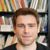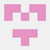# 100 Julia exercises

Here is a Julia version of exercises with solutions which is based on the exercises given here: https://github.com/rougier/numpy-100

#### 1. Import the julia package LinearAlgebra under the name `la`

``````import LinearAlgebra as la #or
import LinearAgebra
const la = LinearAlgebra
``````

#### 2. Print the version of julia package Javis

``````]
status Javis
``````

#### 3. Create a zero vector of size 10 and of type Int64

``````a = zeros(Int64,10)
``````

#### 4. How to find the memory size of any array in bytes

``````sizeof(a)
length(a)*sizeof(eltype(a))
``````

#### 5. How to get the documentation of the Julia zero function from the command line?

``````?zeros
``````

#### 6. Create a Int vector of size 10 but the fifth value which is 1 (in two lines of code)

``````a = zeros(Int,10)
a = 1
``````

#### 7. Create a vector with values ranging from 10 to 49

``````a = [10:49;]
a = collect(10:49)
``````

#### 8. Return the reverse of a vector (first element becomes last)

``````a[end:-1:1] #faster
reverse(a)
reverse!(a) #inplace
``````

#### 9. Create a 3x3 matrix with values ranging from 0 to 8

``````a = reshape(0:8,3,3)
``````

#### 10. Find indices of non-zero elements from [1,2,0,0,4,0]

``````findall(!isequal(0),a)
findall(!iszero,a)
findall(!=(0),a)
``````

#### 11. Create a 3x3 identity matrix

``````using LinearAlgebra
a = I(3)
a = Matrix(1.0I,3,3)
``````

#### 12. Create a 3x3x3 array with random values

``````a = rand(3,3,3) #numbers in the range 0 to 1
b = randn(3,3,3) #Gaussian random numbers
``````

#### 13. Create a 10x10 array with random values and find the minimum and maximum values

``````a = rand(10,10)
minm = minimum(a)
maxm = maximum(a)
minm, maxm = extrema(a)
``````

#### 14. Create a random vector of size 30 and find the mean value

``````using Statistics
a = rand(30) #rand(30,)
b = mean(a)
``````

#### 15. Create a 2d array with 1 on the border and 0 inside

``````a = zeros(5,5)
a[1,:] .= 1
a[end,:] .= 1
a[2:end-1,1] .= 1
a[2:end-1,end] .= 1
a[:,1] .= 1;a[:,end] .= 1 #Last two steps can be replaced by this line
``````

#### 16. How to add a border (filled with 0's) around an existing array?

``````a = rand(5,5)
b = zeros(Float64,size(a,1)+2,size(a,2)+2)# b = zeros(eltype(a), size(a) .+ 1)
b[2:end-1,2:end-1] = a
``````

#### 17. What is the result of the following expression? (★☆☆)

`````` (0 * NaN) >= NaN : false
NaN == NaN : false
NaN > NaN : false
NaN - NaN : NaN
NaN in Set([NaN]) : true
NaN in [NaN] : false
0.3 == 3 * 0.1 : false #floating point rounding error
isapprox(0.3 , 3 * 0.1) : true
``````

#### 18. Create a 5x5 matrix with values 1,2,3,4 just below the diagonal

``````a = Bidiagonal(zeros(5,),collect(1:4),:L)
``````

#### 19. Create a 8x8 matrix and fill it with a checkerboard pattern

``````a = zeros(Int64,8,8)
a[1:2:end,1:2:end] .= 1
a[2:2:end,2:2:end] .= 1 #or
a = [Int(isodd(i+j)) for i in 1:8, j in 1:8]
``````

#### 20. Consider a (6,7,8) shape array, what is the index (x,y,z) of the 100th element?

``````m = 100
n,p,r = 6,7,8
page = Int64(ceil(m/n/p))
col = Int64(ceil((m-(page-1)*n*p)/n))
row = Int64(ceil((m-(page-1)*n*p-(col-1)*n)/n))

CartesianIndices((6,7,8))[m] #builtin command
``````

#### 21. Create a checkerboard 8x8 matrix using the repeat function (★☆☆)

``````repeat([1 0;0 1],4,4)
``````

#### 22. Normalize a 5x5 random matrix (★☆☆)

``````a = randn(5,5)
a = a .- minimum(a)
a = a./maximum(a)
``````

#### 23. Create a custom dtype that describes a color as four unsigned bytes (RGBA) (★☆☆)

``````mutable struct color
R::UInt8
G::UInt8
B::UInt8
A::UInt8
end
``````

#### 24. Multiply a 5x3 matrix by a 3x2 matrix (real matrix product)

``````a = rand(5,3);b = rand(3,2)
c = a*b
``````

#### 25. Given a 1D array, negate all elements which are between 3 and 8, in place. (★☆☆)

``````a = 3;b = 8;
x = rand(1:10,20) # collect(1:10)
x[a .<= x .<= b] .= -x[a .<= x .<= b] #or
x[a .<= x .<= b] .*= -1.
``````

#### 26. What is the output of the following script? (★☆☆) [Python only]

``````
``````

#### 27. Consider an integer vector Z, which of these expressions are legal?

``````Z.^Z #valid
2 << Z >> 2 #not valid
Z <- Z # Z < (-Z)
1im*Z #valid
Z/1/1 #valid,converted to float vector
Z<Z>Z #valid
``````

#### 28. What are the result of the following expressions? (★☆☆)

``````/ : 1x1 Matrix Float64 0.0
 ./  : NaN
//  : MethodError
 .÷  : DivideError
Float64(Int64([NaN])) : MethodError
``````

#### 29. How to round away from zero a float array ? (★☆☆)

``````round.(-4.5, RoundNearestTiesAway)
``````

#### 30. How to find common values between two arrays? (★☆☆)

``````intersect(A,B) #A and B are sets
``````

#### 32. Is the following expressions true? [Python only]★☆☆)

``````np.sqrt(-1) == np.emath.sqrt(-1)
``````

#### 33. How to get the dates of yesterday, today and tomorrow? (★☆☆)

``````using Dates
tod = today()
tom = tod + Day(1)
yes = tod + Day(-1)
``````

#### 34. How to get all the dates corresponding to the month of July 2016? (★★☆)

``````using Dates
mon = collect(Date(2016,7,1):Day(1):Date(2016,7,31))
``````

#### 35. How to compute ((A+B)*(-A/2)) in place (without copy)? (★★☆)

``````A = rand(2,2);B = rand(2,2)
@. A = ((A+B)*(-A/2))
``````

#### 36. Extract the integer part of a random array of positive numbers using 4 different methods (★★☆)

``````A = 10*rand(1,10)
Int64.(trunc.(A))
B = parse.(Int64,[split(string(x),".") for x in A])
Int64.(floor.(A))
Int64.(ceil.(A)) .- 1
``````

#### 37. Create a 5x5 matrix with row values ranging from 0 to 4 (★★☆)

``````a = rand(0:4,5,5)
``````

#### 38. Consider a generator function that generates 10 integers and use it to build an array (★☆☆)

``````a = collect(1:10)
``````

#### 39. Create a vector of size 10 with values ranging from 0 to 1, both excluded (★★☆)

``````a = rand(10)
``````

#### 40. Create a random vector of size 10 and sort it (★★☆)

``````a = rand(10,)
sort!(a)
``````

#### 42. Consider two random array A and B, check if they are equal (★★☆)

``````isequal(A,B)
``````

#### 44. Consider a random 10x2 matrix representing cartesian coordinates, convert them to polar coordinates (★★☆)

``````a = rand(10,2);
ang = angle.(a[:,1] .+ 1im*a[:,2])
r = hypot.(a[:,1] .+ a[:,2])# sqrt.(a[:,1].^2 .+ a[:,2].^2)
p = r.*cis.(ang)  # r.*exp.(1im*ang)
#or
to_polar(x, y) = hypot(x, y) * cis(angle(x+im*y))
[to_polar(x...) for x in eachrow(a)] #or
[to_polar(a[i, 1], a[i, 2]) for i in axes(a, 1)]
``````

#### 45. Create random vector of size 10 and replace the maximum value by 0 (★★☆)

``````a = rand(10)
a[a .== maximum(a)] .= 0
``````

#### 46. Create a structured array with `x` and `y` coordinates covering the [0,1]x[0,1] area (★★☆)

``````mutable struct xy
x:Float64
y:Float64
end
``````

#### 47. Given two arrays, X and Y, construct the Cauchy matrix C (Cij =1/(xi - yj)) (★★☆)

``````X = rand(5);Y = rand(5);
C = [1/(xi-yi) for xi in X, yi in Y]#or
C = 1 ./ (X .- Y')
``````

#### 48. Print the minimum and maximum representable value for each Julia scalar type

``````typemin()
typemax()
``````

#### 49. How to print all the values of an array? (★★☆)

``````print(a)
display(a)
foreach(println, a) #prints full array
``````

#### 50. How to find the closest value (to a given scalar) in a vector? (★★☆)

``````a = rand(10)
x = 0.5 #given scalar
b = abs.(a .- x)
a[findmin(b)]
``````

I will update remaining exercises in due course.Logan Kilpatrick

It would be great to get these setup and linked on GitHub as well! Let me know if you want to put them in the Julia Community GitHub organization.I think #44 can be done more simply:

``````using LinearAlgebra: norm
a = rand(10, 2)
mapreduce(vcat, eachrow(a)) do r
[norm(r) atan(r...)]
end
``````

Similarly for #50:

``````A = rand(10)
x = 0.5
findmin(a -> abs(a - x), A)
``````

(or `minimum(a -> abs(a - x), A)` if you just want the value of `abs(a - x)` as in your solution)